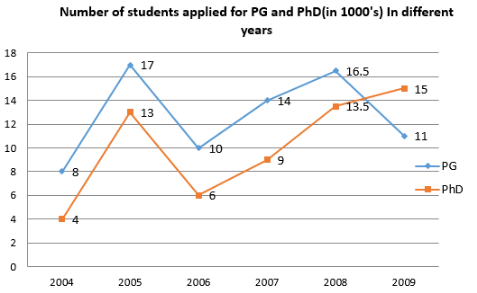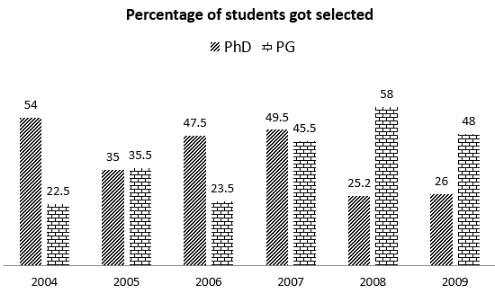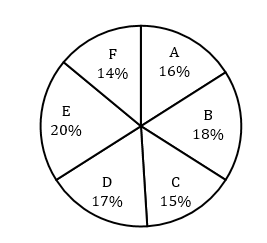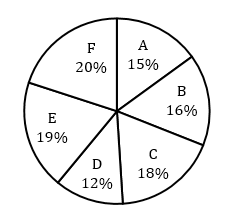## Data Interpretation For SBI PO : Set – 13

(1-5): Read the following graph carefully and answer the questions given below: Delhi University offers two courses PG and PhD. The information regarding number of students applied for these two courses and among how many got selected from year 2004-2009 are shown by the graph given below:1.What is the respective ratio of the percentage increase/decrease in the students got selected for PG in 2005 over Year 2004 to the percentage increase/decrease in the number of students applied for PhD in year 2008 over year 2007.

(a) 47 : 10

(b) 5 : 23

(c) 41 : 17

(d) 27 : 7

(e) 26 : 9

1) (a)

2. Average number of students got selected for PhD program is by what percent more/less the average number of students applied for PG programs.

(a) 72% less

(b) 72% more

(c) 82% less

(d) 82% more

(e) 77% more

2) (a)

3. Which year shows the highest quantum difference between the number of students applied and got selected for PhD programs.

(a) 2004

(b) 2005

(c) 2006

(d) 2008

(e) 2009

3) (e)

4. Ratio of Number of students selected in 2005, 2007 and 2009 for PhD course to number of students applied in 2004, 2006 and 2008 for same course is ?

(a) 2389 : 4980

(b) 2581 : 4700

(c) 2679 : 4321

(d) 2471 : 5321

(e) None of the above

4) (b)

5. In PG program which year shows highest percentage increase/decrease in number of student selected over previous year?

(a) 2005

(b) 2006

(c) 2007

(d) 2008

(e) 2009

5) (a)

(6-10) Study the following pie-chart and table carefully to answer these questions

State wise details of adult population of a country.

Graduate and above, total number = 24 LakhsUpto XII Std. Pass, Total number = 32 lakhsMALE and FEMALE RATIO

 STAETS GRADUATE AND ABOVE UPTO XII STANDARD PASS M:F M:F A 7:5 7:9 B 5:3 3:5 C 5:4 4:5 D 9:8 5:7 E 9;7 9:10 F 4:3 3:2

6.What is the difference between graduate male population and XII standard male population from state A?

(a) 24000

(b) 14000

(c) 28000

(d) 36000

(e) None of these

6) B

7. What is the ratio between graduate female population of state E and standard XII female population of state D respectively?

(a) 7 : 5

(b) 5 : 7

(c) 16 : 15

(d) 15 : 16

(e) None of these

7) D

=   2.24lakhs

Ratio = 2.1 : 2.24 = 210 : 224 = 15:16

8. Graduate female population of state C is what per cent of the standard XII female population of the state?

(a) 40

(b) 62.5

(c) 50

(d) 52.5

(e) None of these

8) C

Required % = 100 = 50%

9. Class XII male population of state C is what per cent of the total standard XII population of all the state together?

(a) 8%

(b) 12%

(c) 11%

(d) 9%

(e) None of these

9) A

XII std female population of C = 32 = 2.56 lakhs

Required % = 100 = 8%

10. What is the ratio between the graduate male population of state E and standard XII female population of that state respectively?

(a) 28 : 35

(b) 35 : 28

(c) 32 : 45

(d) 45 : 32

(e) None of these